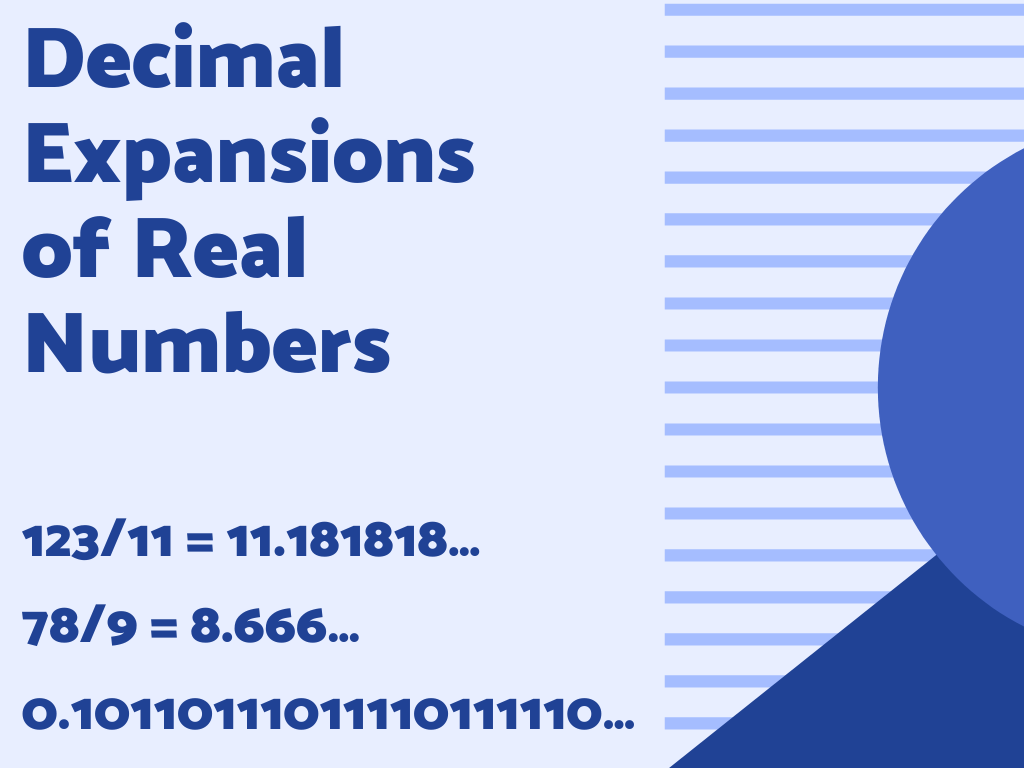## Decimal Expansions of Real Numbers Class 9th

As we know that collections of all rational numbers and irrational numbers are known as real numbers. In this section, we shall study decimal expansions of real numbers means decimal expansions of rational numbers and irrational numbers. First, we start with rational numbers. Decimal Expansions of Rational Numbers Rational numbers are in the form of …